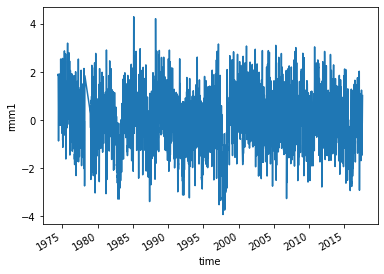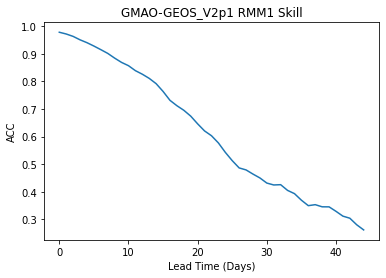You can run this notebook in a live sessionor view it on Github.

# Calculate skill of a MJO Index of SubX model GEOS_V2p1 as function of daily lead time#

In this example, we demonstrate:

1. How to remotely access data from the Subseasonal Experiment (SubX) hindcast database and set it up to be used in `climpred`.

2. How to calculate the Anomaly Correlation Coefficient (ACC) using daily data with `climpred`

3. How to calculate and plot historical forecast skill of the real-time multivariate MJO (RMM) indices as function of lead time.

## The Subseasonal Experiment (SubX)#

Further information on SubX is available from Pegion et al.  and the SubX project website.

The SubX public database is hosted on the International Research Institute for Climate and Society (IRI) data server http://iridl.ldeo.columbia.edu/SOURCES/.Models/.SubX/.

Since the SubX data server is accessed via this notebook, the time for the notebook to run may is several minutes and will vary depending on the speed that data can be downloaded. This is a large dataset, so please be patient. If you prefer to download SubX data locally, scripts are available from https://github.com/kpegion/SubX.

## Definitions#

RMM

Two indices (RMM1 and RMM2) are used to represent the MJO. Together they define the MJO based on 8 phases and can represent both the phase and amplitude of the MJO . This example uses the observed RMM1 provided by Matthew Wheeler at the Center for Australian Weather and Climate Research. It is the version of the indices in which interannual variability has not been removed.

Skill of RMM

Traditionally, the skill of the RMM is calculated as a bivariate correlation encompassing the skill of the two indices together . Currently, `climpred` does not have the functionality to calculate the bivariate correlation, thus the anomaly correlation coefficient for RMM1 index is calculated here as a demonstration. The bivariate correlation metric will be added in a future version of `climpred`.

```# linting
```
```---------------------------------------------------------------------------
ModuleNotFoundError                       Traceback (most recent call last)
Cell In, line 2
1 # linting

File ~/checkouts/readthedocs.org/user_builds/climpred/conda/latest/lib/python3.9/site-packages/IPython/core/interactiveshell.py:2456, in InteractiveShell.run_line_magic(self, magic_name, line, _stack_depth)
2454     kwargs['local_ns'] = self.get_local_scope(stack_depth)
2455 with self.builtin_trap:
-> 2456     result = fn(*args, **kwargs)
2458 # The code below prevents the output from being displayed
2459 # when using magics with decorator @output_can_be_silenced
2460 # when the last Python token in the expression is a ';'.
2461 if getattr(fn, magic.MAGIC_OUTPUT_CAN_BE_SILENCED, False):

31 if not module_str:
32     raise UsageError('Missing module name.')

69 """Load an IPython extension by its module name.
70
73 function, or None if it succeeded.
74 """
75 try:
77 except ModuleNotFoundError:
78     if module_str in BUILTINS_EXTS:

89 with self.shell.builtin_trap:
90     if module_str not in sys.modules:
---> 91         mod = import_module(module_str)
92     mod = sys.modules[module_str]

125             break
126         level += 1
--> 127 return _bootstrap._gcd_import(name[level:], package, level)

File <frozen importlib._bootstrap>:1030, in _gcd_import(name, package, level)

File <frozen importlib._bootstrap>:1007, in _find_and_load(name, import_)

File <frozen importlib._bootstrap>:984, in _find_and_load_unlocked(name, import_)

ModuleNotFoundError: No module named 'nb_black'
```
```import warnings

import matplotlib.pyplot as plt

import xarray as xr
import pandas as pd
import numpy as np

from climpred import HindcastEnsemble
import climpred

warnings.filterwarnings("ignore")
```

```obsds = climpred.tutorial.load_dataset("RMM-INTERANN-OBS")[["rmm1"]].dropna(
"time"
)  # Get rid of missing times.
```
```obsds["rmm1"].plot()
```
```[<matplotlib.lines.Line2D at 0x14d9e5b20>]
```Read the SubX RMM1 data for the `GMAO-GEOS_V2p1` model from the SubX data server. It is important to note that the SubX data contains weekly initialized forecasts where the `init` day varies by model. SubX data may have all NaNs for initial dates in which a model does not make a forecast, thus we apply `dropna` over the `S=init` dimension when `how=all` data for a given `S=init` is missing. This can be slow, but allows the rest of the calculations to go more quickly.

Note that we ran the `dropna` operation offline and then uploaded the post-processed SubX dataset to the `climpred-data` repo for the purposes of this demo. This is how you can do this manually:

```url = 'http://iridl.ldeo.columbia.edu/SOURCES/.Models/.SubX/.GMAO/.GEOS_V2p1/.hindcast/.RMM/.RMM1/dods/'
fcstds = xr.open_dataset(url, decode_times=False, chunks={'S': 1, 'L': 45}).dropna(dim='S',how='all')
```
```fcstds = climpred.tutorial.load_dataset("GMAO-GEOS-RMM1", decode_times=False)
fcstds
```
```<xarray.Dataset>
Dimensions:  (S: 510, M: 4, L: 45)
Coordinates:
* S        (S) float32 1.424e+04 1.425e+04 1.426e+04 ... 2.044e+04 2.045e+04
* M        (M) float32 1.0 2.0 3.0 4.0
* L        (L) float32 0.5 1.5 2.5 3.5 4.5 5.5 ... 40.5 41.5 42.5 43.5 44.5
Data variables:
RMM1     (S, M, L) float32 -0.03237 0.1051 0.1946 ... -0.1499 0.08326 0.1389
Attributes:
Conventions:  IRIDL```

The SubX data dimensions correspond to the following `climpred` dimension definitions: `X=lon`,`L=lead`,`Y=lat`,`M=member`, `S=init`. We will rename the dimensions to their `climpred` names.

```fcstds = fcstds.rename({"S": "init", "L": "lead", "M": "member", "RMM1": "rmm1"})
```

Let’s make sure that the `lead` dimension is set properly for `climpred`. SubX data stores `leads` as 0.5, 1.5, 2.5, etc, which correspond to 0, 1, 2, … days since initialization. We will change the `lead` to be integers starting with zero.

```fcstds["lead"] = (fcstds["lead"] - 0.5).astype("int")
```

Now we need to make sure that the `init` dimension is set properly for `climpred`. We use the `xarray` convenience function `decode_cf` to convert the numeric time into datetimes. It recognizes that this is on a 360 day calendar.

```fcstds = xr.decode_cf(fcstds, decode_times=True)
```

`climpred` also requires that `lead` dimension has an attribute called `units` indicating what time units the `lead` is assocated with. Options are: `years`, `seasons`, `months`, `weeks`, `pentads`, `days`, `hours`, `minutes`, or `seconds`. For the SubX data, the `lead` `units` are `days`.

```fcstds["lead"].attrs = {"units": "days"}
```

Create the `HindcastEnsemble` object and `HindcastEnsemble.add_observations()`.

```hindcast = HindcastEnsemble(fcstds).add_observations(obsds)
hindcast
```

Calculate the Anomaly Correlation Coefficient (ACC) `climpred.metrics._pearson_r()`

```skill = hindcast.verify(
metric="acc", comparison="e2o", dim="init", alignment="maximize"
)
skill
```
```<xarray.Dataset>
Coordinates:
* lead     (lead) int64 0 1 2 3 4 5 6 7 8 9 ... 35 36 37 38 39 40 41 42 43 44
skill    <U11 'initialized'
Data variables:
rmm1     (lead) float64 0.9782 0.9719 0.9632 0.9508 ... 0.304 0.2801 0.2616
Attributes:
Conventions:                   IRIDL
skill_calculated_by_function:  HindcastEnsemble.verify()
number_of_initializations:     510
number_of_members:             4
alignment:                     maximize
metric:                        pearson_r
comparison:                    e2o
dim:                           init
reference:                     []```

Plot the skill as a function of lead time

```skill["rmm1"].plot()
plt.title("GMAO-GEOS_V2p1 RMM1 Skill")
```Text(0, 0.5, 'ACC')